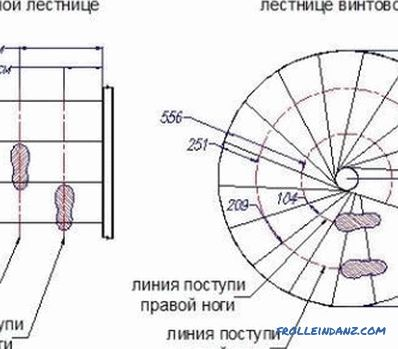# How to calculate the length of the rafters for the roof: formula, calculation table

When constructing the roof, various parameters must be considered. Among them, the most important are the length and pitch of the truss system. It is also important to know how to calculate the length of the rafter. Take into account the expected load, the angle of the slope. Without taking them into account, it is impossible to begin work, since the data obtained will not be reliable. Of course, there are many standard values ​​that can be used. But there are a number of nuances that can have a critical impact on the strength and reliability of the structure.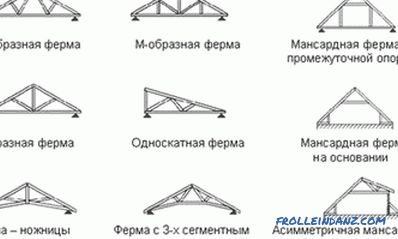Roof construction options for civil engineering.

## What should be taken into account when calculating?

For the length of rafters to be chosen correctly, it is necessary to take into account numerous parameters during the calculations. If you violate certain rules, the roof can simply collapse. These parameters should include:

• type of roof used;
• size and total roof area;
• roofing material.

The complexity of the calculation of the roof system depends largely on what form the future roof will have. The calculation of the truss for a simple gable or complex multi-gable roof will be carried out differently. Do hip, broken roofs need to know not only the size and shape, but also what exactly the design will be enhanced. It is necessary, in addition to truss legs, to use struts, crossbars, sleepers, etc., otherwise the design may collapse under its own weight.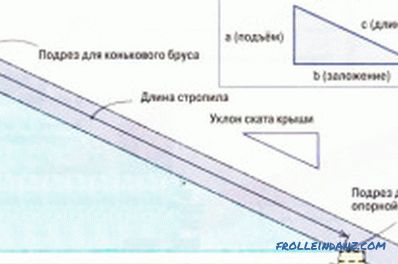The formula for calculating wooden trusses.

Taking into account the total area of ​​the future roof is important for any building structure, since the choice of material depends on the expected loads . It often happens that the builders decide to save and take thin beams for the construction of the structure. Where it leads? At the first winter, the roof will develop under its own weight and weight of snow. In order to prevent the occurrence of such a situation, it is necessary to immediately calculate what kind of load will be provided. It is important to choose the right beam and its cross section. Today, a variety of materials are used as a roof covering, in many respects they are selected for the type of roof. Overlaying soft roofs can only be used for flat roofs, as is the case with PVC membranes and roofing material. Lathing is selected taking into account the future roofing. For the calculation it is necessary to take into account what the material will be, whether there are requirements to the base. For metal tiles, it is necessary to use a batten of battens, which run in 60 cm steps. For a flexible tile, an already solid batten is required. For installation fit sheets of OSB, plywood, chipboard or other material. For natural ceramic tiles, the weight of which is significant, it is necessary to use reinforcement, but this already applies not only to the batten, but also to the truss system. If you do not take into account the weight of ceramics, the roof can collapse under its own weight.

## How is the length of the rafters calculated?

To calculate the length of rafter legs, a simple formula is used that has been well known by many since school times. The structure of the rafters is an isosceles triangle, the angles of which depend on the slope. Such a triangle should be divided into 2 equal parts, getting the same figures. Then you can apply the formula for a right triangle, and the corners are known, there is the value of one leg. The formula has the form c = √ (a2 + b2), where: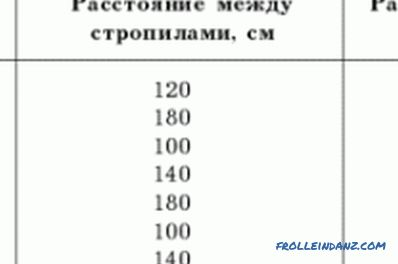Table of calculation of the truss system.

• s is the hypotenuse, that is, the desired length of one truss;
• a is a straight line that divides an isosceles triangle (the projection of the truss system) by 2 .

If it is necessary to calculate the angle of installation of the truss system, i.e. the convergence of 2 truss legs in the ridge, the formula is used: tg (A) = a / b. To obtain the angle itself, the resulting value should be multiplied by 2, since the large triangle was divided into 2 small ones. Since the sum of all angles must be equal to 180 °, it is necessary to subtract from the total value the right angle (90 °) and the resulting value, thus obtaining the angle of installation of the rafters relative to the wall. To calculate the length of the rafters is enough knowledge of the Pythagorean theorem. It must be remembered that the length will depend on other indicators, which include snow and wind loads, the type of roofing material, the shape and size of the roof itself. Special formulas are also used here. To calculate the snow loads, the formula S = µ is applied. Sg, where:

• S is the snow loads required;
• µ is the coefficient (the roof slope must be taken into account, expressed in degrees);
• Sg is the standard snow load which depends on the region.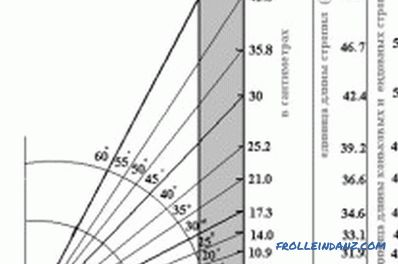Scheme of the length of rafters on the angle of inclination of the roof.

The coefficient is determined very simply, if the slope is less than or equal to 30 °, then µ = 1. If the angle is greater than or equal to 60 °, then µ = 0. Snow regulatory load is determined using SNiP 2. 01. 07-85.For the calculation, the formula Wm = Wo is used. K. C, where:

• Wm is the desired quantity;
• Wo is the standard value of the wind pressure in a particular region;
• K is the coefficient of change in wind pressure;
• С - special aerodynamic effect.

The normative value is determined by the tabular data given in SNiP 2. 01. 07-85.

To calculate the length of rafters, it is important to know which roofing will be used. This value is closely connected with the shape of the roof, the angle of the slope. Such moments should be foreseen in advance, as for some types of roofing materials the slope is of fundamental importance. During the calculation of the load, we must not forget that the crate also has weight. If the roofing material is too massive, the roof system will be difficult. We'll have to provide special support elements.

The calculation of the length of the rafters and the distance between them is an important step in all calculations. The data is very dependent on many parameters, they include the step between the legs of the structure, the cross section of the beam and so on. During operation it is recommended to use ready-made tabular data. There are a number of specialized programs that allow you to perform all the calculations quickly and correctly.

•